# Which Way SPRA?¶

## UCLA, 16 September 2018¶

Dr. Ravindra claims that PRA works.

The only evidence he provided is that it is widely used.

That demonstrates there is a market for PRA, but not that it works--other than to provide employment (and a fig leaf).

Fallacies do not cease to be fallacies because they become fashions.

—G.K. Chesterton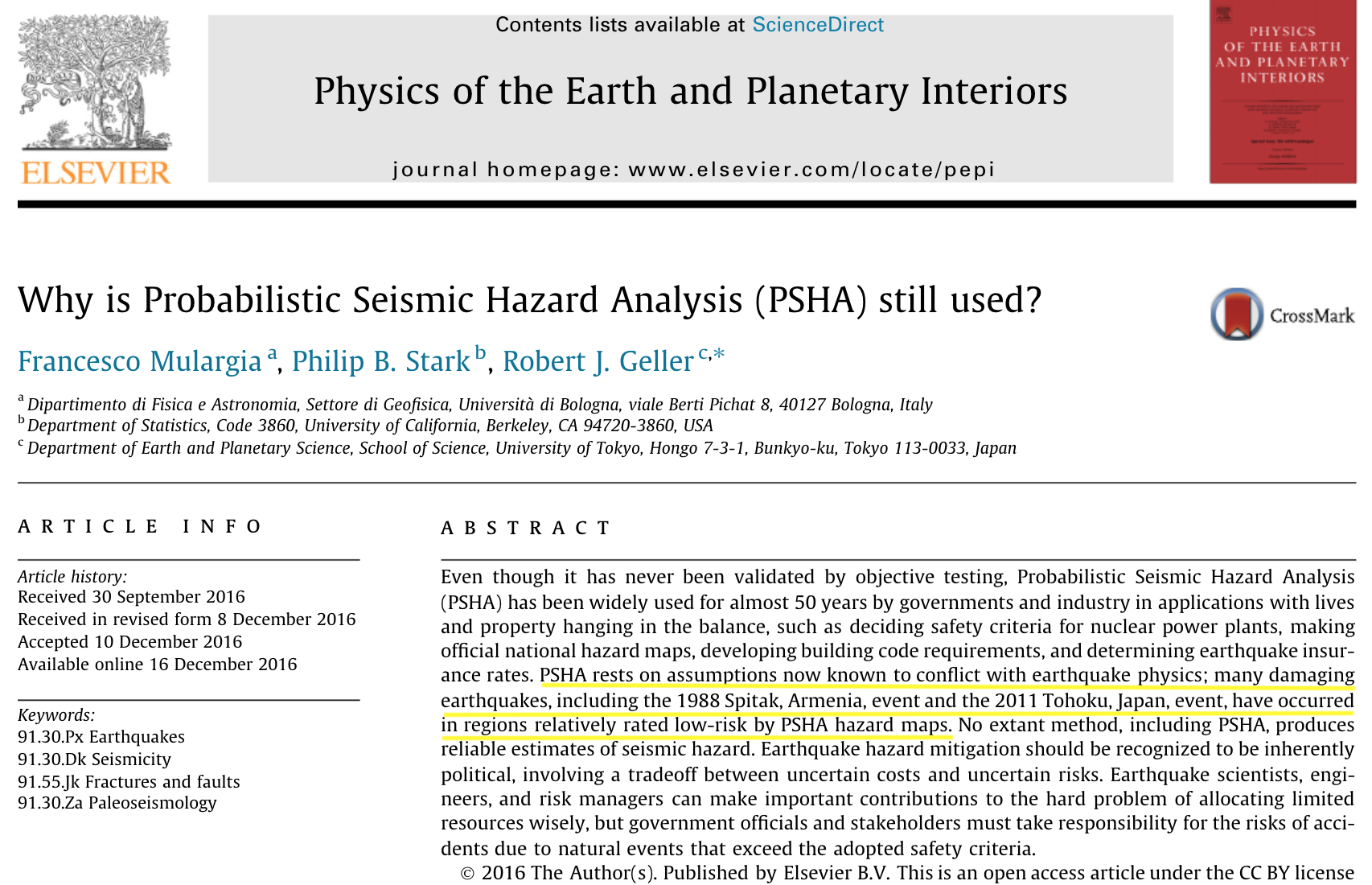• Physics--not much
• lab experiments on rupture/fracture have limited applicability
• theoretical models simplistic; don't predict main empirical aspects
• Phenomenology
• clustering in space and time
• "characteristic earthquakes" & "recurrence times" inconsistent w/ data
• Gutenberg-Richter relationship (to about M7.6)
• Omori's law

### Does it make sense to talk about "probability of an earthquake" or "exceedance probability"?¶

It is inappropriate to be concerned about mice when there are tigers abroad.

—George Box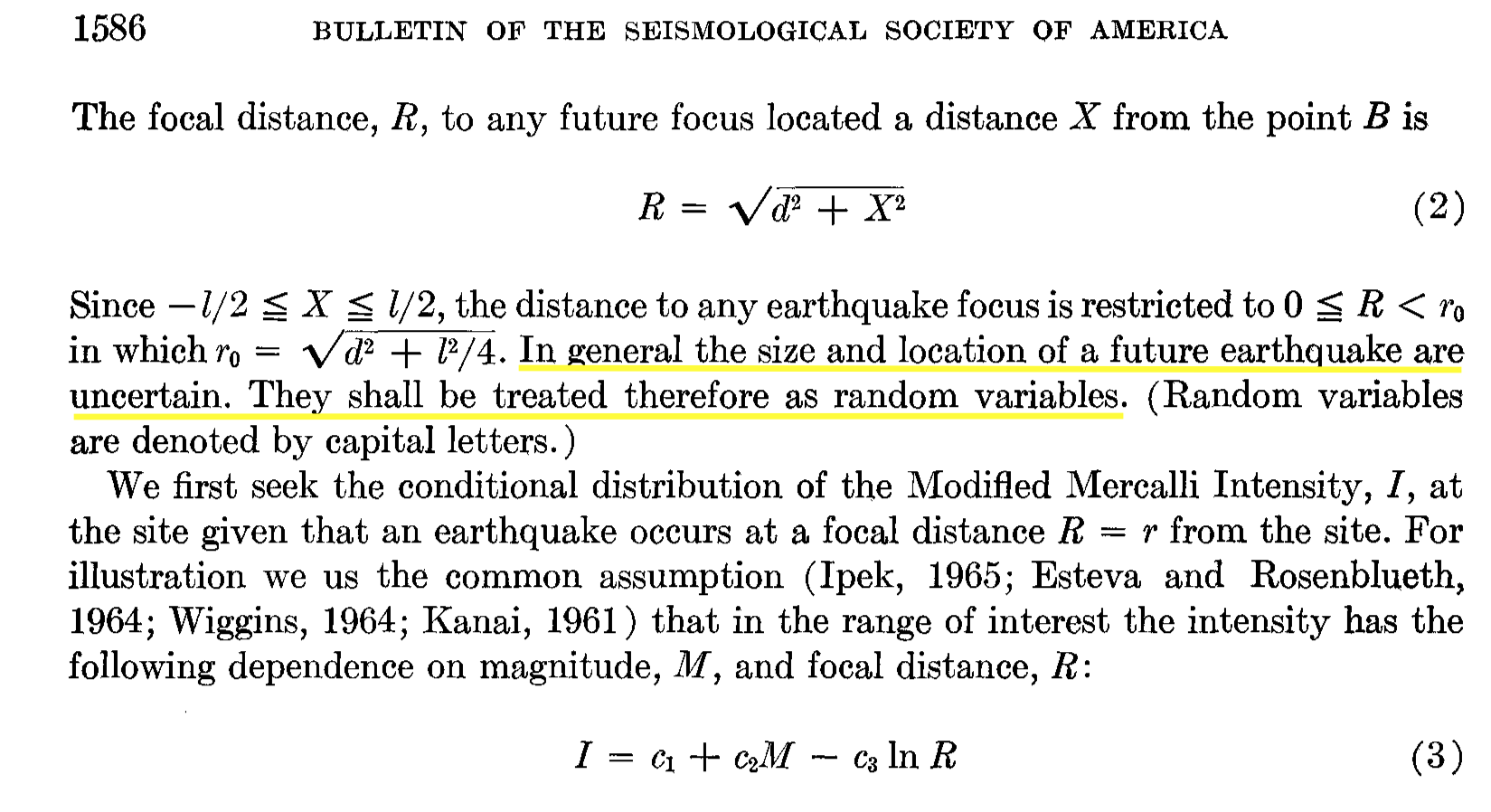|

### PSHA¶

Model event location & mag as a marked stochastic process with known parameters.

Model ground motion in a given place as a random variable, given event location and mag.

Then,

probability of a given level of ground movement in a given place is the integral (over space and magnitude) of the conditional probability of that level of movement given that there's an event of a particular magnitude in a particular place, times the probability that there's an event of a particular magnitude in that place

• That events occur at random is an assumption

• That ground motion resulting from an event of a given magnitude in a given place is random is an assumption

• Standard argument:

• M8 events happen in a given region about once a century.
• Therefore, the chance of an M8 event in the region is about 1% per year.

### Rates versus probabilities¶

• In a series of trials, if each trial has the same probability $p$ of success, and the trials are independent, then the rate of successes converges (in probability) to $p$. Law of Large Numbers
• If a finite series of trials has an empirical rate $p$ of success, that says nothing about whether the trials are random.
• If the trials are random and have the same chance of success, the empirical rate is an estimate of the chance of success.
• If the trials are random and have the same chance of success and the dependence of the trials is known (e.g., the trials are independent), can quantify the uncertainty of the estimate.
• Even if the trials are random, if the system is nonstationary and/or if the number of trials observed is small, the past rate is not a reliable estimate of future probability
• Gutenberg-Richter relation & Omori's law describe rates not probability distributions
• 1 M8 per ~100y does not mean ~1% chance per year.

### How does probability enter a scientific problem?¶

• underlying phenomenon is random (quantum physics)

• deliberate randomization (randomized experiments, random sampling)

• subjective probability

• model that's supposed to describe the phenomenon (thermodynamics)

• in what sense?
• to what level of accuracy?
• description v. prediction v. predicting effect of intervention
• testable to desired level of accuracy?
• metaphor: phenomenon behaves "as if random"

"Probability" often invoked reflexively to represent uncertainty (since postwar period)

Not all uncertainty can be represented by a probability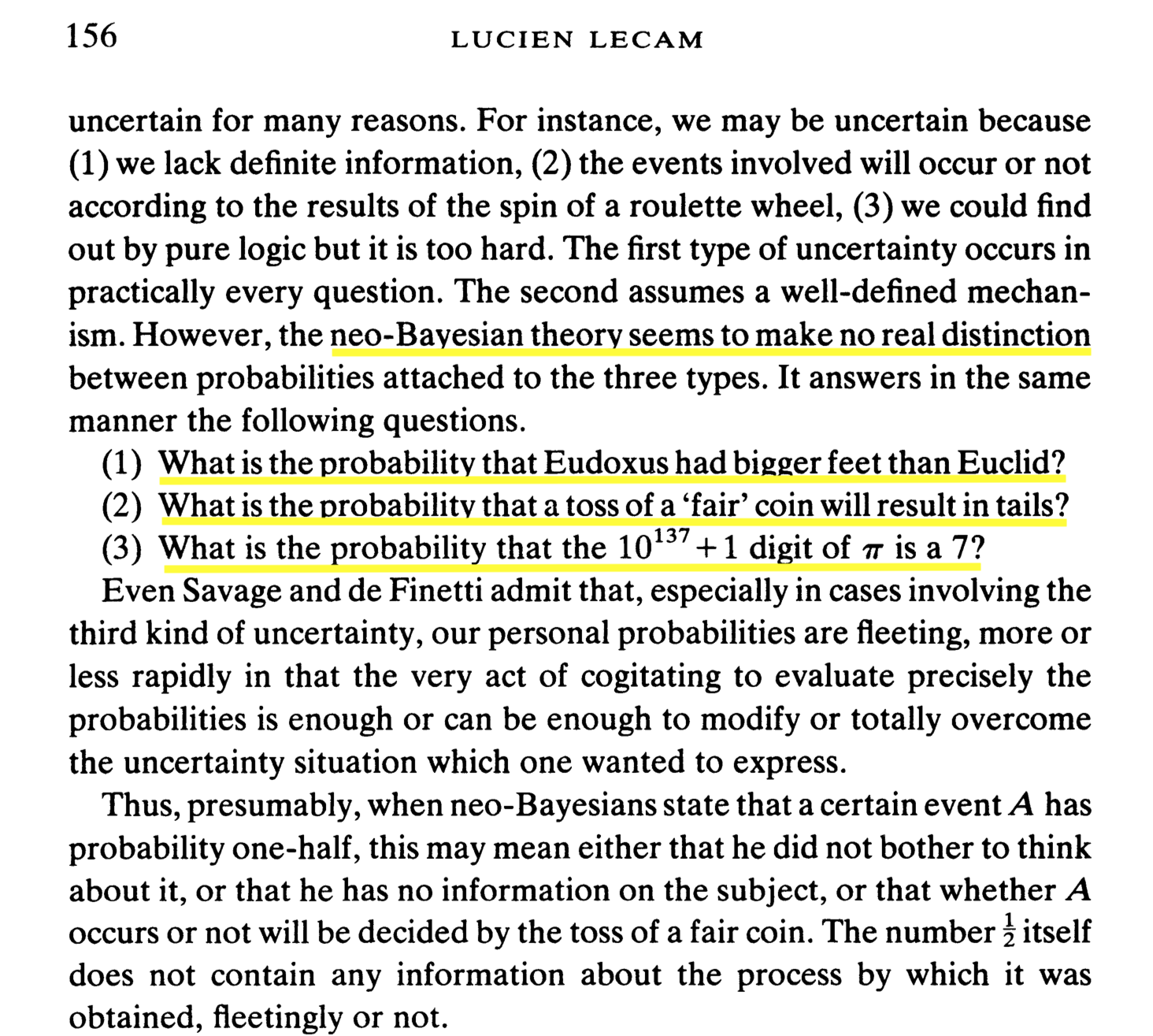### Do Monte Carlo simulations estimate real-world probabilities?¶

• Monte Carlo is a way to substitute computing for calculation--not for observation

• doesn't reveal anything that was not already an assumption in the calculation

• distribution of the output results entirely from input assumptions

• randomness is an assumption, not a conclusion; distribution is an assumption, not a conclusion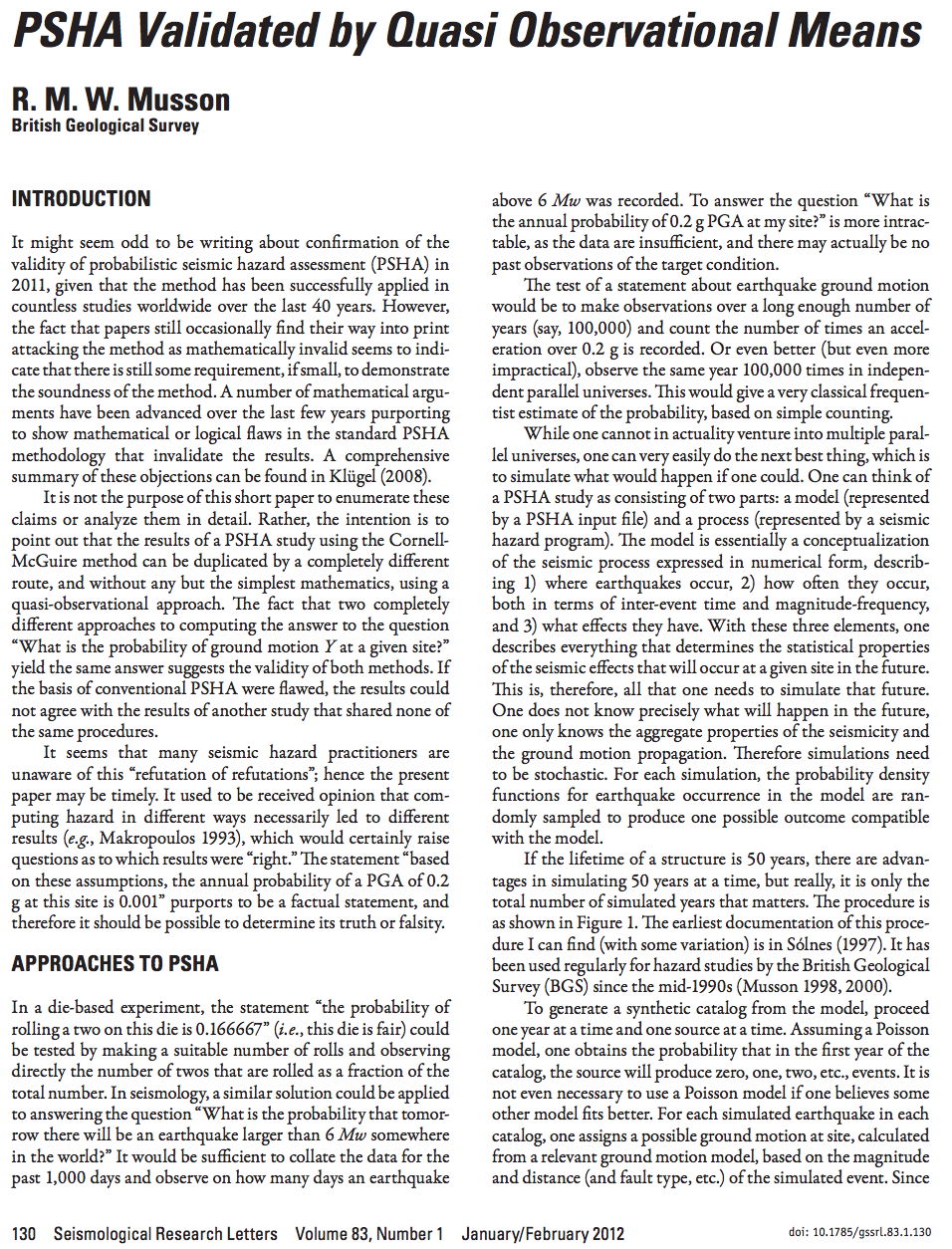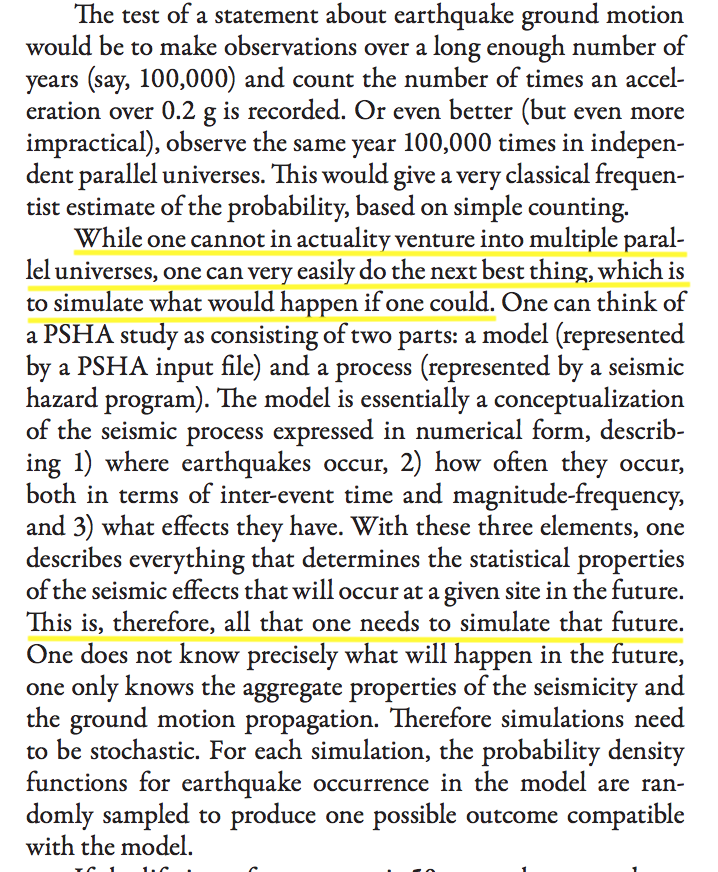Circular!

Assumes seismicity is random with known probability distribution, simulates from that distribution, and claims that simulation output validates the assumptions.

### Fault trees¶

• became popular post-WWII for aerospace, nuclear power, Cold War politics
• might make sense for engineered systems w/ well understood components (although assumptions about independence, etc., can have big impacts; viz. talk by Dr. Budnitz)
• false confidence for complex systems that are not understood, e.g., seismogenesis

### Earthquake casinos¶

• stochastic seismic models amount to saying there's an "earthquake deck"

• turn over one card per period. If the card has a number, that's the size quake you get.

• journals full of arguments about how many "8"s in the deck, whether the deck is fully shuffled, whether cards are replaced and re-shuffled after dealing, etc.

• just a metaphor!

### Earthquake terrorism¶

• Why not say earthquakes are like terrorist bombings?
• don't know where or when
• know they will be large enough to kill
• know some places are "likely targets"
• no probabilities

#### What would make the casino metaphor apt?¶

1. the physics of earthquakes might be stochastic. But it isn't.
1. stochastic models might provide a compact, accurate description of earthquake phenomenology. But it doesn't.
1. stochastic models might be useful for predicting future seismicity. But they aren't (Poisson, Gamma renewal, ETAS)

## A better question¶

Instead of pretending we can quantify seismic hazard, we'd be better off saying,

If we're willing to spend \\$X to reduce risk, what should we spend it on?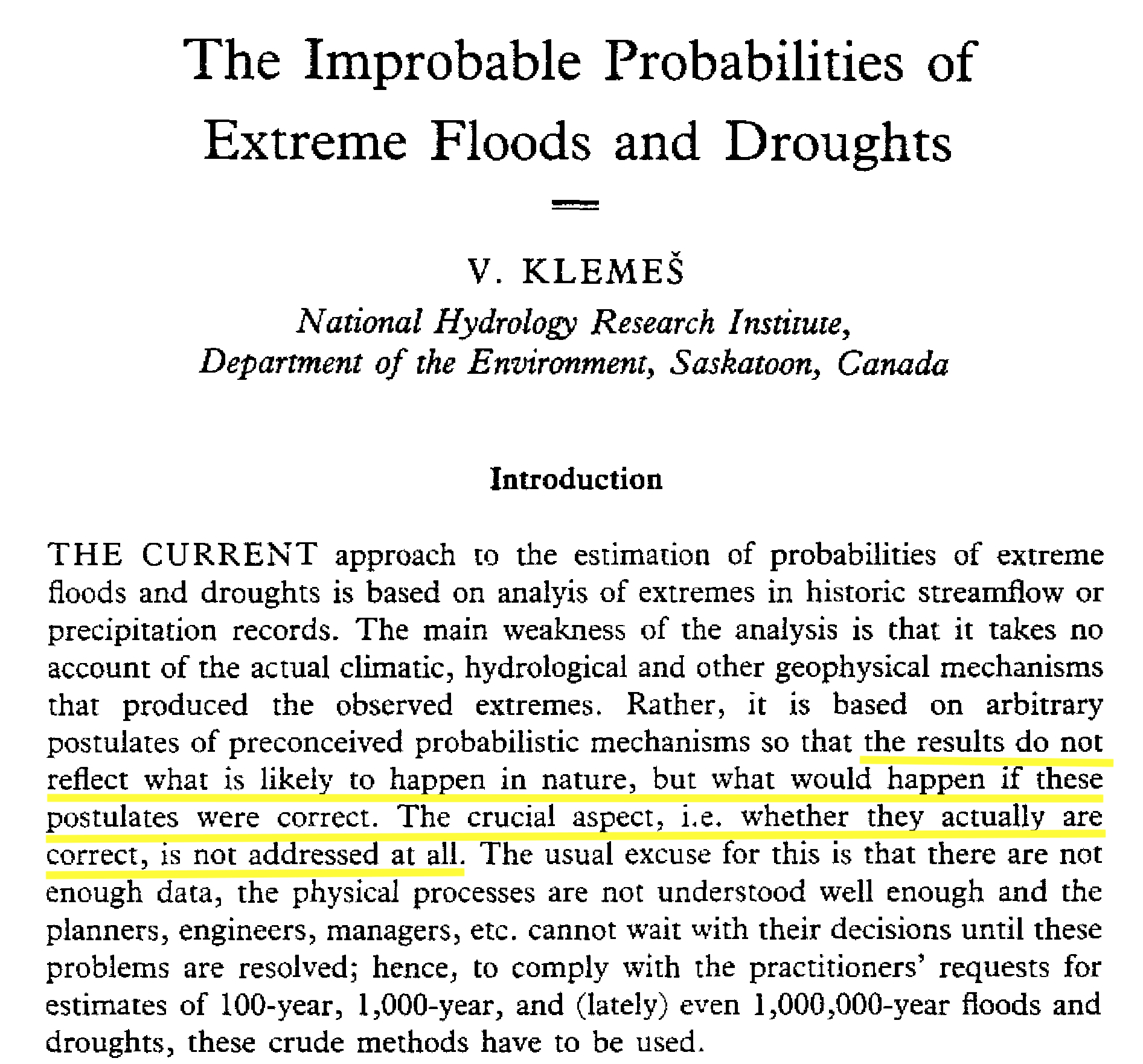If we are uncritical we shall always find what we want: we shall look for, and find, confirmations, and we shall look away from, and not see, whatever might be dangerous to our pet theories. In this way it is only too easy to obtain what appears to be overwhelming evidence in favor of a theory which, if approached critically, would have been refuted.

—Karl Popper